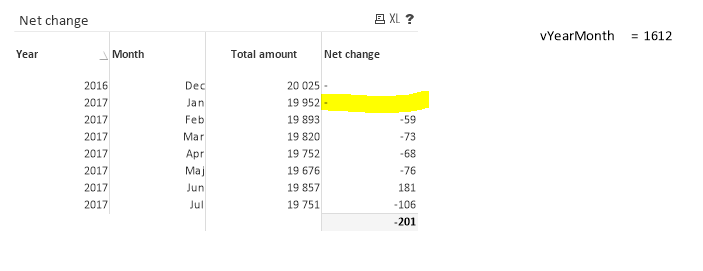# QlikView App Development

Discussion Board for collaboration related to QlikView App Development.

Announcements
Modernize Your QlikView Deployment webinar, Nov. 3rd. REGISTER
cancel
Showing results for
Did you mean:
HighlightedCreator

## Problem calculating net change month to month

Hi

Having problem with my expression trying to calculate net change month to mounth. I am getting null value on first and second row, first row I can understand but why the second row?Total amout = Just calculates the number of ID's for a certain period/month.

count({<Period={">=\$(=\$(vYearMonth))"}>}DISTINCT ID)

Net change = the difference month to month, i.e. april= april - march, 19752-19820= -68

count({<Period={">=\$(=\$(vYearMonth))"}>}DISTINCT ID) - above(count({<Period={">=\$(=\$(vYearMonth))"}>}DISTINCT ID))

I am stuck and can't figure this out.

Any help appreciated

Thanks

5 Replies
HighlightedSpecialist II

Hi,

The Problem seems to be in  Variable Declaration 1701 is not taking the Above Value as 1612, where as 1702 for feb is taking 1701 as Above Properly.

Can you Check. Or share a sample app

HighlightedMVP

would you be able to share the sample?

HighlightedCreator

Hi

I have created a sample app that illustrates the problem: Test.qvwHighlightedMVP

try this

num(count({<Period={">=\$(=\$(vYearMonth))"}>}DISTINCT ID) - above(total count({<Period={">=\$(=\$(vYearMonth))"}>}DISTINCT ID)),'# ##0','.',' ')

HighlightedCreator

Fantastic !!!

Using "total" solved the problem.

Thank you